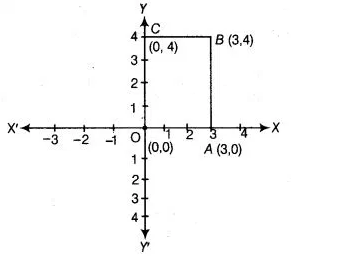# On plotting the points 0(0, 0), A(3, 0), 5(3, 4), C(0, 4)`
Question:

On plotting the points 0(0, 0), A(3, 0), 5(3, 4), C(0, 4) and joining OA, AB, BC and CO. Which of the following figure is obtained?

(a) Square

(b) Rectangle

(c) Trapezium

(d) Rhombus

Solution:

(b) Here, point 0 (0, 0) is the origin. A(3, 0) lies on positive direction of X-axis, B (3, 4) lies

in 1st quadrant and C (0, 4) lines on positive direction of Y-axis. On joining OA AB, BC

and CO the figure obtained is a rectangle.